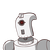# 5x + 7y = 17 if the x= 3 find the value of y Maths 10th standard

5x + 7y = 17 if the x= 3 find the value of y
Maths 10th standard

### 1 thought on “5x + 7y = 17 if the x= 3 find the value of y <br /> Maths 10th standard”

1.The value of y is 2/7.

Step-by-step explanation:

5×3 + 7y = 17

7y = 2

y =2/7